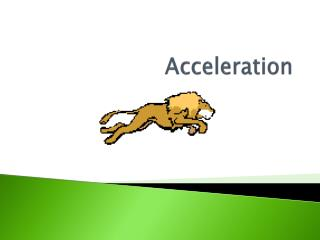DownloadDownload PresentationAcceleration

# Acceleration

Télécharger la présentation## Acceleration

- - - - - - - - - - - - - - - - - - - - - - - - - - - E N D - - - - - - - - - - - - - - - - - - - - - - - - - - -
##### Presentation Transcript

1. Acceleration

2. Acceleration • Acceleration is a measure of the change in velocity doing a period of time. • Acceleration changes when velocity changes due to: increasing speed, decreasing speed, or changing direction. • Acceleration = final speed – initial speed time

3. Practice Problems • If the canoe accelerates from 0 m/s to 0.8 m/s in 0.2 s, what is the acceleration? • Susan drops a ball, and 2 s later the ball has a speed of 20 m/s. What is the ball’s acceleration? • Dave is traveling at 20 m/s in a car when the car hits a tree. Dave comes to a complete stop in 0.4 s. What is Dave’s acceleration?

4. An ostrich running at 25 m/s hits a fence and comes to a complete stop in 2 s. What was the ostrich’s acceleration? • What is the acceleration of a speedboat that accelerates from 40 m/s to 60 m/s in only 2 s? • Steve puts on his roller blades and skates through the park in 50 s at 5 m/s. What is his acceleration?